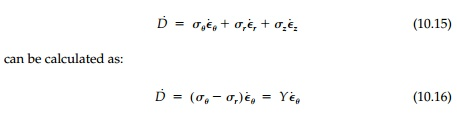Home | | Mechanics of Solids | Limit Load by a ‚ÄúWork‚Äù Calculation

# Limit Load by a ‚ÄúWork‚Äù Calculation

The plastic collapse or limit load can be calculated from equilibrium or by a work calculation based on an assumed mechanism of collapse without first evaluating the elastic and plastic behavior of the structure.

Limit Load by a ‚ÄúWork‚Äù Calculation

The plastic collapse or limit load can be calculated from equilibrium or by a work calculation based on an assumed mechanism of collapse without first evaluating the elastic and plastic behavior of the structure. For the thick ring it can be obtained directly from integrating the equilibrium equation since at collapseComputing this collapse load (without reference to any partially elastic behavior leading to it) by equating internal to external plastic work incre-ments is often easier and is quite different conceptually since there is no need to consider stress distribution or even equilibrium. This calculation can be thought of in terms of three steps:

1.  Guess a collapse mechanism, i.e., formulate the geometry of incre-mental deformation of a plausible failure mechanism.

2.  For this incremental deformation, integrate the ‚Äúwork consumed‚Äù (i.e., energy) in plastic deformation over the body.

3.  Equate this ‚Äúinternal plastic work‚Äù to the external work done by the limit load.

The first step is to describe the geometry of incremental deformation so we can use the flow rule for plastic deformation increments discussed in Section 10.1. For the thick ring:is expressed in terms of the radial expansion of the inner circumference, let c a uÀôa and thereforeThis is a state of pure shear deformation on planes at 45o to the radius.Thus the total dissipation of work per unit length will be:Finally, equating this to the incremental external work done by pL as the ring expands by uÀôaThis is exactly the same result, but it is obtained by considering only the geometry of the deformation of the collapse mechanism, the normality rule, and the yield condition. In fact, no consideration of equilibrium was neces-sary or, for that matter, was the distribution of stress in the ring required.

Study Material, Lecturing Notes, Assignment, Reference, Wiki description explanation, brief detail
Civil : Principles of Solid Mechanics : Concepts of Plasticity : Limit Load by a ‚ÄúWork‚Äù Calculation |

Related Topics